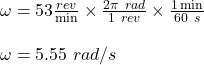Question

A ball, 1.8 kg, is attached to the end of a rope and spun in a horizontal circle above a student’s head. As the student rotates the ball in a horizontal clockwise circle their lab partner counts 53 rotations in one minute. What is the ball’s angular velocity in radians per second?

1.The angular speed of the ball in radians per second is 5.55 rad/s.

Explanation:

Given;

mass of the ball, m = 1.8 kg

number of the ball’s rotation per minute, n = 53 RPM

The angular speed of the ball in radians per second is calculated as follows;Therefore, the angular speed of the ball in radians per second is 5.55 rad/s.